Learn US

# Sine Function

2nd Sep '205 views7 mins read

 1 Introduction 2 What is a Sine Function? 3 Properties of Sine 4 Sine Graph 5 Range, Periodicity, other Properties 6 Importance of Trigonometry in Real Life 7 Summary 8 Frequently Asked Questions (FAQs)

September 2, 2020

## Introduction

The sine function was first found as jyā and koṭi-jyā functions utilized in the Gupta dynasty when Indian astronomy (Aryabhatiya, Surya Siddhanta) was established, via translation from Sanskrit to Arabic and then from Arabic to Latin. The word "sine" (Latin "sinus") comes from a Latin mistranslation by Robert of Chester of the Arabic jiba, which may be a transliteration of the Sanskrit word for half the chord, jya-ardha.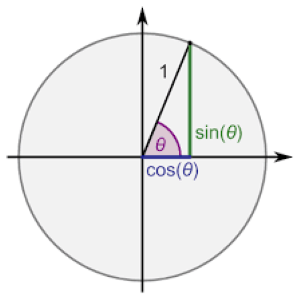Here is a detailed lesson on this sine function is given which will include the following topics:
•    Definition
•    Trigonometry Formula
•    Properties
•    Sine Graph
•    Range, Periodicity, and other Properties
•    Application of Trigonometry in real life
•    FAQs

## What is a Sine Function?

The sine function can be defined as the ratio of the length of the opposite side to that of the hypotenuse in a right-angled triangle. For any triangle, for example, ABC, with an angle a, the sine function will be: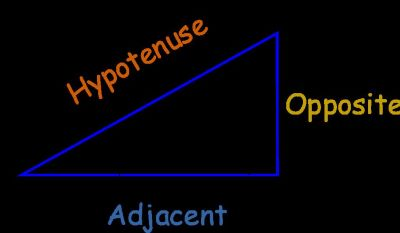Sine Function Formula: From the above diagram, it is known that Sinθ = Opposite/ Hypotenuse.
Now, the Trigonometry formula for sine can be written as:
Sinθ = a/h where a is the side opposite to the angle being referred to and h is the length of the hypotenuse.

Sine Values Table

 Sine Degrees Sine Values Sine 0° 0 Sine 30° 1/2 Sine 45° 1/√2 Sine 60° √3/2 Sine 90° 1 Sine 120° √3/2 Sine 150° 1/2 Sine 180° 0 Sine 270° -1 Sine 360° 0

## Properties of Sine

The sine function has both values positive or negative depending upon the quadrants. In the above table, it is seen that sine 270° is negative while sine 90° is positive. For the sine function, the value is positive for the first and second quadrants while it is negative for values in third and fourth quadrants.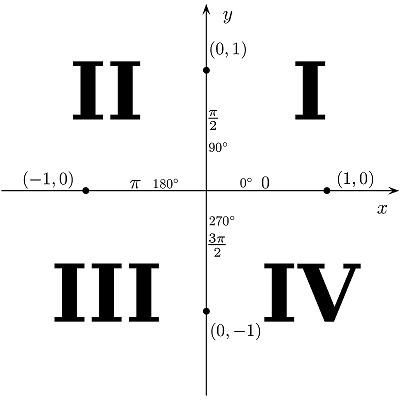## Sine Graph

The sine function graph is as shown: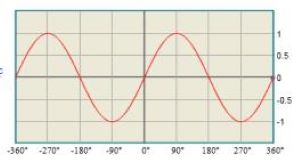When the sine of an angle is graphed against the angle, the resulting sine function graph is a shape almost like that above, called a sine wave or sine curve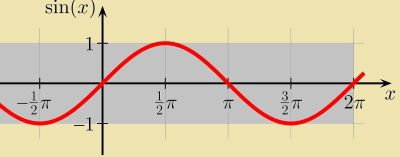The sine graph looks like the image given above. The sine graph or sinusoidal graph is a periodic and constant up and down graph which has a periodicity of 360 deg or 2π which basically implies that it repeats every 360 degrees i.e. at 2π.

Sine Rule: One major application of sine can be seen in sine rule. It is used not, only for right angled triangle but any kind. Using it, we can solve any type of triangle easily.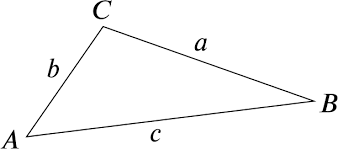According to the sine rule:
When we divide side let us say, a, by the sine of its opposite angle A
the ratio is equal to if side b is divided by the sine of its opposite angle B,
which is also equal to side c divided by the sine of opposite angle C
Or
a/sin A = b/sin B = c/sin C

## Range, Periodicity, other Properties

The sine inverse function is used to measure the angle of a right-angled triangle from the given ratios. The inverse of sine is said to be arcsin, asin, or sin-1.

Let us assume a right triangle is taken with sides 1, √3, and 2. Now, to calculate angle a, the sine function can be used as-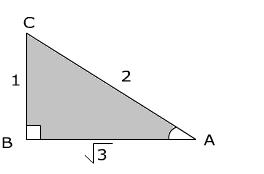Now, sine (a) = opposite/hypotenuse i.e. ½.
The angle A can be computed using the arcsine function.
sin−1(½) = a
That implies that the value of angle “a” is 30°
The graph repeats itself after 2π, which suggests the functions are periodic with a period of 2π. What is a periodic function?

A periodic function is a function that has a specific horizontal shift, P, results in a function equal to the original function: f (x + P) = f (x) for all values of x within the domain of f.
This occurrence of horizontal shift and repeating of graph leads us to call the smallest such horizontal shift with P > 0 the period of the function.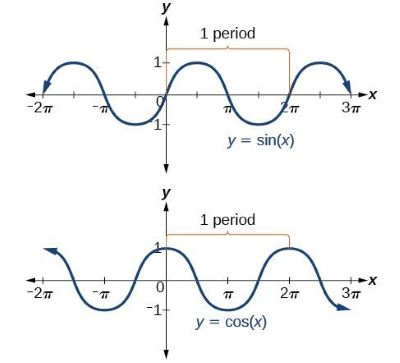Looking at the sine functions on a domain centered at the y-axis helps bring out its symmetries. As we can see in the Figure above, the sine function is symmetric about the origin. So the domain of sine is all real numbers. The range of sine is [-1,1].

Domain of Sine: R

Range of Sine: [-1,1]

The other trigonometric functions that we determined from the unit circle show that the sine function is an odd function because of sin(−x) =−sin x sin(−x) = −sin x. Now we can see this odd function property from the graph.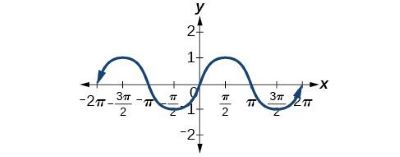## Importance of Trigonometry in Real Life

The techniques in trigonometry are used for finding its importance in navigation particularly satellite systems and astronomy, naval and aviation industries, oceanography, land surveying, and in cartography (creation of maps). Now, these are the scientific applications of the concepts in trigonometry of class 10, but most of the math we study would seem (on the surface) to have little real-life application. So, is trigonometry important in our day to day activities?Let's look into these areas where this science finds use in our daily activities and how we can use this to resolve problems we might encounter. Although it is unlikely that we will ever need to directly apply a trigonometric function in solving a practical issue, the fundamental background of science finds usage in an area that is a passion for several - music!As you may be aware sound travels in waves and this pattern though not as regular as a sine function, is still useful in developing computer music. Since a computer does not function like us in the sense it cannot hear and comprehend music like we do, it represents music mathematically by its constituent sound waves. This also means that sound engineers and technologists who work on the advances in computer music and even many music composers have to familiarize themselves and apply the basics of trigonometry.

## Summary

• The sine is a function of trigonometry of an angle. The sine of an acute angle can be defined with reference to a right triangle: it is the ratio of the length of the side that is opposite that angle to the length of the longest side of the triangle (the hypotenuse). Sinθ = Opposite/ Hypotenuse
• The sine functions have several distinct characteristics:
• They are periodic functions with a period of 2π.
• The domain of each function is (−∞, ∞) and the range is [−1,1].
• The graph of y = sin x is symmetric about the origin because it’s an odd function.
• y = cos x is an even function which implies it is symmetric about the y-axis.

Trigonometry of class 10 finds a perfect partner in modern architecture. The sine function is usually used to model periodic phenomena like sound and light waves, the position and velocity of harmonic oscillators, sunlight intensity and day length, and average temperature variations throughout the year.
Learning math sure makes us smart and adept at solving tricky situations. From tacking brain-teasers and jigsaws to the more complex crises, the appliance of basic laws of math and geometry are many. Not only does math provide a robust strong basis for resolving everyday issues, it undoubtedly helps handle situations with a positive approach.

Written by Priti Jain, Cuemath Teacher

0

1/2

1/√2

√3/2

0

√3/2

## sin 270?

-1

Related Articles
GIVE YOUR CHILD THE CUEMATH EDGE
Access Personalised Math learning through interactive worksheets, gamified concepts and grade-wise courses